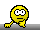## Calculation of time above MIC [🇷 for BE/BA]

Hi HS,

the time above MIC was calculated during the Steady State, which goes from 12 hours to 120 hours. That's why the results differs. (I was struggling with this part as well).

Here the updated code:
 time <- c(0.5, 1, 3, 5, 8, 12, 24, 36, 48, 60,           72, 84, 96, 108, 120, 122, 124) conc <- c(0.64, 0.7, 1.39, 1.35, 0.67, 1.48, 0.32, 2.08, 0.87, 0.93,           1.09, 0.59, 1.01, 0.47, 0.99, 0.62, 0.13) dat  <- data.frame(time, conc) f <- function(dat, th) {   under <- 0   for (i in which(dat$conc < th)) { if (!is.na(dat$conc[i-1])) {         y <- dat$conc[c(i-1,i)] x <- dat$time[c(i-1,i)]         slope <- coef(lm(y~x))         under <- under + x-x-(th-y)/slope       }       if (!is.na(dat$conc[i+1])) { y <- dat$conc[c(i,i+1)]         x <- dat$time[c(i,i+1)] slope <- coef(lm(y~x)) under <- under + (th-y)/slope } } return(under) } th <- 0.517 first <- 12 last <- 120 occupancy <- last-first - f(dat[dat$time >=first & dat\$time <=last, ], th) coverage  <- 100*occupancy/(last-first) cat(" Time point interval:",first,"-",last,"\n",     "Occupancy time:",occupancy,"\n",     "Coverage:",coverage,"%\n") 

AceIng. Helmut Schütz